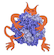IMP  2.3.1 The Integrative Modeling Platform
IMP::core::XYZ Class Reference

A decorator for a particle with x,y,z coordinates. More...

#include <IMP/core/XYZ.h>Inheritance diagram for IMP::core::XYZ:

## Detailed Description

A decorator for a particle with x,y,z coordinates.Using the decorator one can get and set coordinates and modify derivatives.

1 ## \example core/XYZ_Decorator.py
2 # This is a simple example using the XYZDecorator to set the coordinates
3 # of some particles and compute the distance between them.
4
5 import IMP
6 import IMP.core
7 import IMP.algebra
8
11 # add x,y,z coordinates to the particle
14 # add slots for the coordinates, but leave them uninitialized
16 # set the coordinate values
17 d1.set_coordinates(IMP.algebra.Vector3D(3, 4, 5))
18
19 # return the distance between the two points described by the decorated
20 # particles
21 print IMP.core.get_distance(d0, d1)
XYZR
Examples:
core/simple.cpp.

Definition at line 30 of file XYZ.h.

## Public Member Functions

XYZ (::IMP::kernel::Model *m,::IMP::kernel::ParticleIndex id)

void add_to_derivative (int i, Float v, DerivativeAccumulator &d)
Add something to the derivative of the ith coordinate. More...

void add_to_derivatives (const algebra::Vector3D &v, DerivativeAccumulator &d)
Add something to the derivative of the coordinates. More...

Float get_coordinate (int i) const
Get the ith coordinate. More...

const algebra::Vector3Dget_coordinates () const
Convert it to a vector. More...

bool get_coordinates_are_optimized () const
Get whether the coordinates are optimized. More...

Float get_derivative (int i) const
Get the ith coordinate derivative. More...

algebra::Vector3D get_derivatives () const
Get the vector of derivatives. More...

algebra::Vector3D get_vector_to (const XYZ &b) const
Get the vector from this particle to another. More...

Float get_x () const

Float get_y () const

Float get_z () const

void set_coordinate (unsigned int i, Float v)
set the ith coordinate More...

void set_coordinates (const algebra::Vector3D &v)
set all coordinates from a vector More...

void set_coordinates_are_optimized (bool tf) const
Set whether the coordinates are optimized. More...

void set_x (Float t)

void set_y (Float t)

void set_z (Float t)

void show (std::ostream &out=std::cout) constPublic Member Functions inherited from IMP::kernel::Decorator
bool get_is_valid () const

Modelget_model () const
Returns the Model containing the particle. More...

Particleget_particle () const
Returns the particle decorated by this decorator. More...

ParticleIndex get_particle_index () const
Returns the particle index decorated by this decorator. More...

operator Particle * () const

operator ParticleIndex () const

Particleoperator-> () const

## Static Public Member Functions

static FloatKey get_coordinate_key (unsigned int i)

static bool get_is_setup (const IMP::kernel::ParticleAdaptor &p)

static bool get_is_setup (kernel::Model *m, kernel::ParticleIndex pi)

static const FloatKeysget_xyz_keys ()
Get a vector containing the keys for x,y,z. More...

static XYZ setup_particle (kernel::Model *m, ParticleIndex pi)

static XYZ setup_particle (IMP::kernel::ParticleAdaptor decorator, algebra::Vector3D v)

static XYZ setup_particle (kernel::Model *m, ParticleIndex pi, algebra::Vector3D v)Protected Member Functions inherited from IMP::kernel::Decorator
Decorator (Model *m, ParticleIndex pi)

## Member Function Documentation

 void IMP::core::XYZ::add_to_derivative ( int i, Float v, DerivativeAccumulator & d )

Add something to the derivative of the ith coordinate.

Definition at line 75 of file XYZ.h.Here is the call graph for this function:

 void IMP::core::XYZ::add_to_derivatives ( const algebra::Vector3D & v, DerivativeAccumulator & d )

Add something to the derivative of the coordinates.

Definition at line 79 of file XYZ.h.Here is the call graph for this function:

 Float IMP::core::XYZ::get_coordinate ( int i ) const

Get the ith coordinate.

Definition at line 69 of file XYZ.h.Here is the call graph for this function:

 const algebra::Vector3D& IMP::core::XYZ::get_coordinates ( ) const

Convert it to a vector.

Somewhat suspect based on wanting a Point/Vector differentiation but we don't have points

Definition at line 107 of file XYZ.h.Here is the call graph for this function:

 bool IMP::core::XYZ::get_coordinates_are_optimized ( ) const

Get whether the coordinates are optimized.

Returns
true only if all of them are optimized.

Definition at line 86 of file XYZ.h.Here is the call graph for this function:

 Float IMP::core::XYZ::get_derivative ( int i ) const

Get the ith coordinate derivative.

Definition at line 73 of file XYZ.h.

 algebra::Vector3D IMP::core::XYZ::get_derivatives ( ) const

Get the vector of derivatives.

Somewhat suspect based on wanting a Point/Vector differentiation but we don't have points

Definition at line 114 of file XYZ.h.Here is the call graph for this function:

 algebra::Vector3D IMP::core::XYZ::get_vector_to ( const XYZ & b ) const

Get the vector from this particle to another.

Definition at line 99 of file XYZ.h.Here is the call graph for this function:

 static const FloatKeys& IMP::core::XYZ::get_xyz_keys ( )
static

Get a vector containing the keys for x,y,z.

This is quite handy for initializing movers and things.

 void IMP::core::XYZ::set_coordinate ( unsigned int i, Float v )

set the ith coordinate

Definition at line 58 of file XYZ.h.Here is the call graph for this function:

 void IMP::core::XYZ::set_coordinates ( const algebra::Vector3D & v )

set all coordinates from a vector

Examples:
core/simple.cpp.

Definition at line 62 of file XYZ.h.Here is the call graph for this function:

 void IMP::core::XYZ::set_coordinates_are_optimized ( bool tf ) const

Set whether the coordinates are optimized.

Definition at line 92 of file XYZ.h.Here is the call graph for this function:

 static XYZ IMP::core::XYZ::setup_particle ( kernel::Model * m, ParticleIndex pi )
static

Setup the particle with unspecified coordinates. Setup the particle so it can be used with this decorator.

Examples:
atom/dope_and_excluded_volume.cpp, and core/simple.cpp.

Definition at line 51 of file XYZ.h.

 static XYZ IMP::core::XYZ::setup_particle ( kernel::Model * m, ParticleIndex pi, algebra::Vector3D v )
static

Setup the particle so that it can be used with this decorator

Definition at line 52 of file XYZ.h.

 static XYZ IMP::core::XYZ::setup_particle ( IMP::kernel::ParticleAdaptor decorator, algebra::Vector3D v )
static